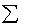previous lesson

F-test

INFERENCE--one nominal and one interval variable

Fisher's F-test: F = mean square variance between groups
---------------------mean square variance within groups

Where: mean square variance = sum of squares
----------------------------------degrees of freedom

Where: between group sum of squares is:B = {[( Yj )2 / nj ]} - [( Y )2 / N ]

Between group degrees of freedom is: d.f.B = k - 1

Within group sum of squares is:w = {[Yj2 - [ (Yj)2/nj ]}

Within group degrees of freedom is: d.f.w = N - K

Where: nj = number in a nominal category

Y = interval variable

k = number of nominal categories

N = total in sample

Assumptions:

Normal distribution of interval variable in whole population

Independent nominal categories

Similar variance in each category

Example:
 Income: Union Non-union 10 2 10 3 5 2 5 3

Q: Can this difference be generalized to the whole population?

A: First, check if assumptions have been met:

1) This class will assume normal distribution because it is difficult to show without SPSS.

2) Assume independent nominal categories as this is also difficult to prove.

3) Similar variance in each category can be proven by Bartlet's test, which is quite involved, therefore assume similar variance in each category unless using SPSS.
 Union Y2 Non Union Y2 10 100 2 4 10 100 3 9 5 25 2 4 5 25 3 9 30=Yu 250= Yu2 10 =Ynu 26 = Ynu2

nu = 4 ; nnu = 4

Total sample: Y = Yu + Ynu = 30 + 10 = 40

N = nu + nnu = 4 + 4 = 8

Between group sum of squares:B = (30)2/4 + (10)2/4 - (40)2/8 = 50

Between group degrees of freedom: K - 1 = 2 - 1 = 1

Within group sum of squares:w = {250 - [(30)2/4} + {26 - [(10)2/4]} = 26

Within group degrees of freedom: N - K = 8 - 2 = 6

 d.f. Sum of Squares Mean Square Variance F Between groups 1 50 50 / 1 = 50 50 / 4.33 = Within groups 6 26 26 / 6 = 4.33 11.54

Interpretation: Look up p-value of F in table in appendix. Although the F-test has two separate tables, interpret the same as for other p-value charts.

p = .05

 d.f.w d.f.B 1 2 3 1 2 3 4 5 6 5.99 7 :

p = .01

 d.f.w d.f.B 1 2 3 1 2 3 4 5 6 13.74 7 :

Can't generalize Can generalize

p > .05 --------p = .05---- p < .05------------ p = .01

-------------------5.99---- F = 11.54 -----------13.74

So, for this example, one can generalize the difference to the whole

population because p < .05.